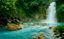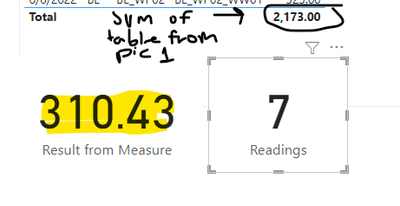cancel
Showing results for
Did you mean:Helper III

## Calculating a sum of multiple locations

Hello,

So, my goal is to calculate the total reading/output of a specific city and compare it to what is required by law. I take the last 90 days of readings to calculate this.

Here's what I want: the average readings by specific equipment, and then the total of the averages (picture attached)Right now, I have a measure that calculates the # of readings in the last 90 days, the sum of the readings, and it divides to find the total average.

Measure =

Var AmountRecorded = SUM(Table[Value]) ---> This is just a column that has the readings
Var Readings = Table[NumOfReadings] ---> This is measure saying countrows that are greater than 0 and not blank
Var MaxDate = MAX(Table[Date])
Return
CALCULATE(
DATESBETWEEN(Table[Date], MaxDate, MaxDate - 90))

The measure above is essentially performing this...It combines all the values and calculates a total average. Instead, I would want a measure that adds 250, 350, and 324 to give me a total "average" of 924.

Please let me know if I need to add any other info for help.

Thanks!

1 ACCEPTED SOLUTIONSolution Sage

Hi,

The below formula should work for your requirment.

Result = SUMX(SUMMARIZE ( 'Table', 'Table'[Equipment] ),CALCULATE( AVERAGE('Table'[Reading])))

Also result should be 250 + 250 + 291 = 891 ( instead of 924)

Thank You,

Regards,Solution Sage

Hi,

The below formula should work for your requirment.

Result = SUMX(SUMMARIZE ( 'Table', 'Table'[Equipment] ),CALCULATE( AVERAGE('Table'[Reading])))

Also result should be 250 + 250 + 291 = 891 ( instead of 924)

Thank You,

Regards,